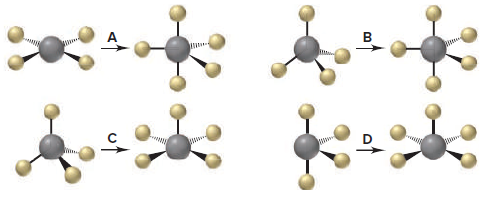# Problem: Consider the following reaction of silicon tetrafluoride:SiF4 + F− ⟶ SiF5−(a) Which depiction below best illustrates the change in molecular shape around Si?

###### FREE Expert Solution

We’re being asked to determine the depiction that best illustrates the change in molecular shape around Si

SiF4 + F ⟶ SiF5

The possible molecular shapes are:For this, we need to do the following steps:

Step 1: Determine the central atom in this molecule.

Step 2: Calculate the total number of valence electrons present.

Step 3: Draw the Lewis structure for the molecule.

Step 4: Determine the shape of the molecule.

99% (227 ratings)###### Problem Details

Consider the following reaction of silicon tetrafluoride:

SiF4 + F ⟶ SiF5

(a) Which depiction below best illustrates the change in molecular shape around Si?Frequently Asked Questions

What scientific concept do you need to know in order to solve this problem?

Our tutors have indicated that to solve this problem you will need to apply the Electron Geometry concept. You can view video lessons to learn Electron Geometry. Or if you need more Electron Geometry practice, you can also practice Electron Geometry practice problems.

What professor is this problem relevant for?

Based on our data, we think this problem is relevant for Professor Wall's class at VT.

What textbook is this problem found in?

Our data indicates that this problem or a close variation was asked in Chemistry: The Molecular Nature of Matter and Change - Silberberg 8th Edition. You can also practice Chemistry: The Molecular Nature of Matter and Change - Silberberg 8th Edition practice problems.